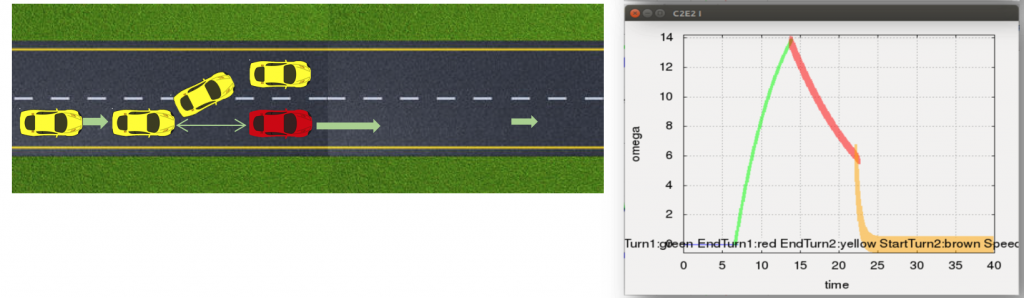# Automatic lane change system (driver assist)

This problem was given as a design problem in a embedded system course. Consider two cars moving on the same lane as shown in the figure below. We have to design a controller for the yellow car to automatically over take the red car and come back to the right lane. Several different control modes are given: slow-down, speed-up, left-turn, right-turn, etc. The dynamics in each mode has certain parameters; also the the transition rules across the modes have parameters. The goal is to design these parameter values so that the overtake maneuver is performed while meeting several requirements.

The model given below fixes the parameter values to numerical constants (so that it can be numerically simulated).

## Dynamics

### MODE 0

$$\left \{ \begin{array}{l} \dot v_x = 0.1 a_x \\ \dot s_x = v_x-2.5 \\ \dot a_x = -0.01 s_x – 0.103 + 0.84 – 0.3 v_x – 0.5 a_x \\ \dot \omega = -2 \omega \\ \dot v_y = -2 v_y \\ \dot s_y = 0.1 v_y \end{array} \right.$$

invariant

$$s_x+10 \lt 0$$

### MODE 1

$$\left \{ \begin{array}{l} \dot v_x = 0.1 a_x \\ \dot s_x = v_x-2.5 \\ \dot a_x = -0.5 v_x + 1.4 -0.5 a_x \\ \dot \omega = 3 – 0.15 \omega + 0.2-0.01 s_y \\ \dot v_y = 7.5 – 0.45 \omega + 0.5 – 0.025 s_y – 0.05 v_y \\ \dot s_y = 0.1 v_y \end{array} \right.$$

invariant

$$s_y \lt 12$$

### MODE 2

$$\left \{ \begin{array}{l} \dot v_x = 0.1 a_x \\ \dot s_x = v_x-2.5 \\ \dot a_x = -0.5 v_x + 1.4 -0.5 a_x \\ \dot \omega = -0.1 \omega + 0.2 – 0.01 s_y \\ \dot v_y = -0.25 \omega + 0.5 – 0.025 s_y – 0.05 v_y \\ \dot s_y = 0.1 v_y \end{array} \right.$$

invariant

$$v_y \gt 0.05$$

### MODE 3

$$\left \{ \begin{array}{l} \dot v_x = 0.1 a_x \\ \dot s_x = v_x-2.5 \\ \dot a_x =-0.5 v_x+1.4-0.5 a_x \\ \dot \omega = -0.1 \omega + 0.2 – 0.01 s_y \\ \dot v_y =-0.25 \omega + 0.5 – 0.025 s_y – 0.05 v_y \\ \dot s_y = 0.1 v_y \end{array} \right.$$

invariant

$$v_y+0.05 \lt 0.$$

### MODE 4

$$\left \{ \begin{array}{l} \dot v_x = 0.1 a_x \\ \dot s_x = v_x-2.5 \\ \dot a_x =-0.5 v_x+1.4-0.5 a_x \\ \dot \omega = -3 – 0.15 \omega + 0.2-0.01 s_y \\ \dot v_y = -7.5 – 0.45 \omega + 0.5 – 0.025 s_y – 0.05 v_y \\ \dot s_y = 0.1 v_y \end{array} \right.$$

invariant

$$s_y \gt 3.5$$

### MODE 5

$$\left \{ \begin{array}{l} \dot v_x = 0.1 a_x \\ \dot s_x = v_x-2.5 \\ \dot a_x = -0.01 s_x – 0.103 + 0.84 – 0.3 v_x – 0.5 a_x \\ \dot \omega = -2 \omega \\ \dot v_y = -2 v_y \\ \dot s_y = 0.1 v_y \end{array} \right.$$

invariant

$$s_x \lt 10$$

### MODE 6

$$\left \{ \begin{array}{l} \dot v_x = 0.1 a_x \\ \dot s_x = v_x-2.5 \\ \dot a_x =-0.5 v_x+1.4-0.5 a_x \\ \dot \omega = -2 \omega \\ \dot v_y = -2 v_y \\ \dot s_y = 0.1 v_y \end{array} \right.$$

## Requirements

The requirements bound the minimum separation between the cars, maximum acceleration of the yellow car; maximum lateral displacement of the car, etc. Please see details in the attached file.

## Analysis with C2E2

This first example shows a scenario where C2E2 proves that the lateral displacement experienced by the yellow car (with the given controller) is within the required range. Tha is, it does not cross over into the shoulder.Reachtube computation shows that the lateral displacement (sy) is bounded within acceptable range.

The next example is executed with different parameter values for which C2E2 finds a “bug” that causes the car to overshoot into the shoulder.

Link to the example file: TotalMotion40s.hyxml